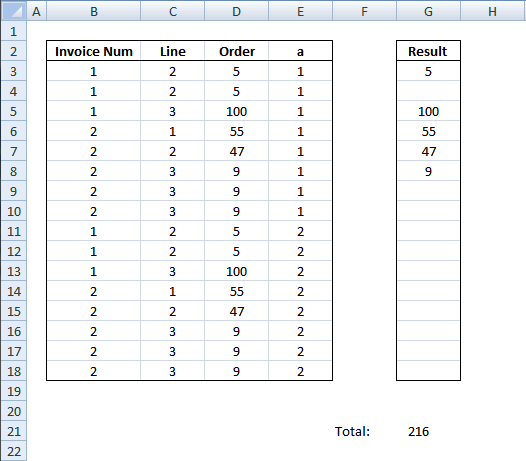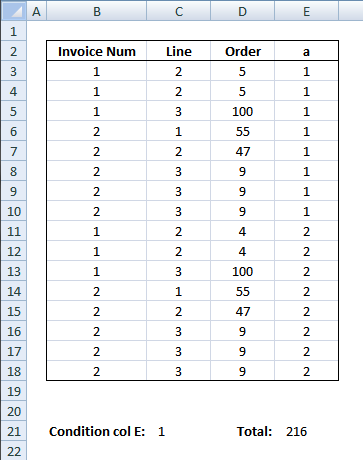Author: Oscar Cronquist Article last updated on January 19, 2019Question:

I have a long table The key is actually col B&C BUT…sometime there are few rows with same key (like rows 3:4 or rows 8:10). I'd like to sum data in column D and to consider same key rows as one row.

Desired result 216

Can I also add condition that Column E=1 ?

I highly recommend a pivot table for this task, it is extremely fast which is good if you have lots of data to work with. This article demonstrates a formula that returns a total based on a condition.Formula in cell E21:

=SUMPRODUCT(--(\$E\$3:\$E\$18=\$C\$21), \$D\$3:\$D\$18, 1/COUNTIFS(\$B\$3:\$B\$18, \$B\$3:\$B\$18, \$C\$3:\$C\$18, \$C\$3:\$C\$18, \$D\$3:\$D\$18, \$D\$3:\$D\$18, \$E\$3:\$E\$18, \$E\$3:\$E\$18))

This formula removes duplicate records and sums values in col D.

### Explaining the formula in cell E21

To simplify the explanation I am replacing cell references with named ranges. The formula becomes:

=SUMPRODUCT(--(ColE=\$C\$21), ColD, 1/COUNTIFS(ColB, ColB, ColC, ColC, ColD, ColD, ColE, ColE))

Named Ranges
ColB -  \$B\$3:\$B\$18
ColC -  \$C\$3:\$C\$18
ColD -  \$D\$3:\$D\$18
ColE -  \$E\$3:\$E\$18

#### Step 1 - Filter records equal to condition in cell E21

The equal sign lets you compare the values in column E with the condition in cell C21, this is a logical expression and the result is either TRUE or FALSE (boolean values), however, the SUMPRODUCT function can't work with boolean values. We need to convert the TRUE and FALSe to their numerical equivalents. TRUE = 1 and FALSE = 0 (zero).

--(ColE=\$C\$21)

--({1; 1; 1; 1; 1; 1; 1; 1; 2; 2; 2; 2; 2; 2; 2; 2}=1)

becomes

--({TRUE; TRUE; TRUE; TRUE; TRUE; TRUE; TRUE; TRUE; FALSE; FALSE; FALSE; FALSE; FALSE; FALSE; FALSE; FALSE})

Sumproduct can´t calculate TRUE/FALSE values. Let´s convert values to 1 and 0.

--({TRUE; TRUE; TRUE; TRUE; TRUE; TRUE; TRUE; TRUE; FALSE; FALSE; FALSE; FALSE; FALSE; FALSE; FALSE; FALSE})

becomes

{1; 1; 1; 1; 1; 1; 1; 1; 0; 0; 0; 0; 0; 0; 0; 0}

#### Step 2 - Create an array with values from col D

ColD

becomes

{5; 5; 100; 55; 47; 9; 9; 9; 4; 4; 100; 55; 47; 9; 9; 9}

#### Step 3 - Count duplicate records

The COUNTIFS function calculates the number of cells across multiple ranges that equals all given conditions.

1/COUNTIFS(ColB, ColB, ColC, ColC, ColD, ColD, ColE, ColE)

becomes

1/{2; 2; 1; 1; 1; 3; 3; 3; 2; 2; 1; 1; 1; 3; 3; 3}

and returns

{0,5; 0,5; 1; 1; 1; 0,333333333333333; 0,333333333333333; 0,333333333333333; 0,5; 0,5; 1; 1; 1; 0,333333333333333; 0,333333333333333; 0,333333333333333}

#### Step 4 - Return the sum of the products of the corresponding arrays

=SUMPRODUCT(--(ColE=\$C\$21), ColD, 1/COUNTIFS(ColB, ColB, ColC, ColC, ColD, ColD, ColE, ColE))

becomes

=SUMPRODUCT({1; 1; 1; 1; 1; 1; 1; 1; 0; 0; 0; 0; 0; 0; 0; 0}, {5; 5; 100; 55; 47; 9; 9; 9; 4; 4; 100; 55; 47; 9; 9; 9}, {0,5; 0,5; 1; 1; 1; 0,333333333333333; 0,333333333333333; 0,333333333333333; 0,5; 0,5; 1; 1; 1; 0,333333333333333; 0,333333333333333; 0,333333333333333})

returns 216 in cell E21.